I.5.6bcd

8/14/2021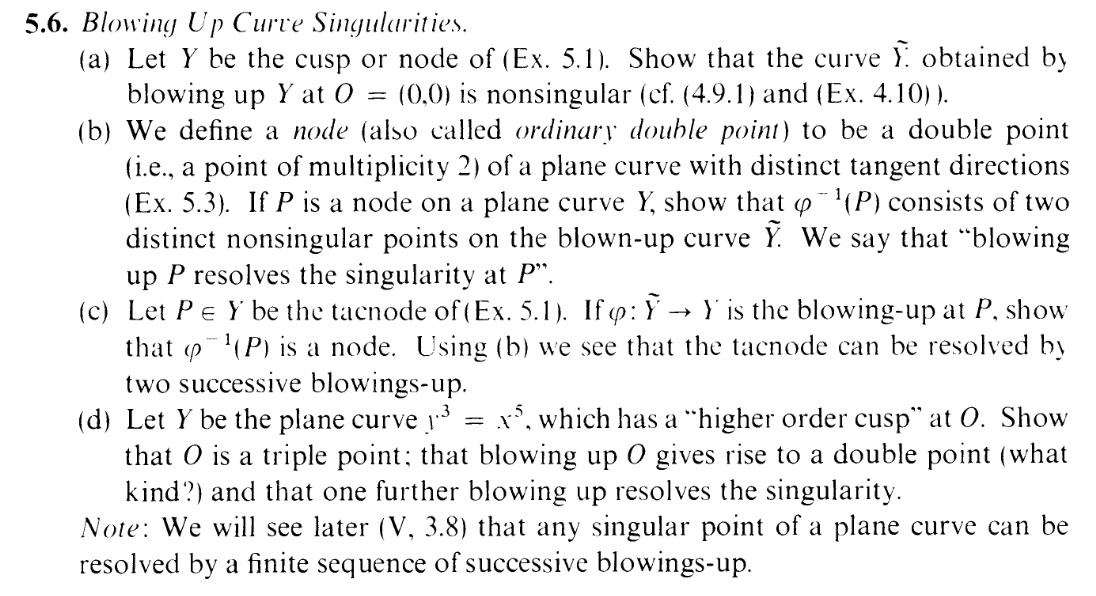HAHAHAHAHAHAHAHAHAHAHAHAHAHAHAHAHAHAHAHAHAHAHAHAHAHAHAHAHAHAHAHAHAHAHAHAHAHAHAHAHAHAHA HAHAHAHAHAHAHAHAHAHAHAHAHAHAHAHAHAHAHAHAHAHAHAHAHAHAHAHAHAHAHAHAHAHAHAHAHAHAHAHAHAHAHA HAHAHAHAHAHAHAHAHAHAHAHAHAHAHAHAHAHAHAHAHAHAHAHAHAHAHAHAHAHAHAHAHAHAHAHAHAHAHAHAHAHAHA HAHAHAHAHAHAHAHAHAHAHAHAHAHAHAHAHAHAHAHAHAHAHAHAHAHAHAHAHAHAHAHAHAHAHAHAHAHAHAHAHAHAHA HAHAHAHAHAHAHAHAHAHAHAHAHAHAHAHAHAHAHAHAHAHAHAHAHAHAHAHAHAHAHAHAHAHAHAHAHAHAHAHAHAHAHA HAHAHAHAHAHAHAHAHAHAHAHAHAHAHAHAHAHAHAHAHAHAHAHAHAHAHAHAHAHAHAHAHAHAHAHAHAHAHAHAHAHAHA HAHAHAHAHAHAHAHAHAHAHAHAHAHAHAHAHAHAHAHAHAHAHAHAHAHAHAHAHAHAHAHAHAHAHAHAHAHAHAHAHAHAHA HAHAHAHAHAHAHAHAHAHAHAHAHAHAHAHAHAHAHAHAHAHAHAHAHAHAHAHAHAHAHAHAHAHAHAHAHAHAHAHAHAHAHA

MATHEMATICS. M-A-T-H-E-M-A-T-I-C-S. What the fuck is that? YOU'RE FLYING. YOU'RE FLYING OVER 120 ACRES. WEEEEEEEEEEEEEE. To think you used to live off scraps. To think you used to be some fucking nomad, trafficked by that pure scum of a human. That one house you squatted for few months in, ugh. You danced around the pressure points of those warped wood slats like you were in a musical, so as not to wake up that scumbag with a creak. And God, those nights spent under a lean-to tarp facing the wrong way. What a joy it is to be under sturdy shingles! What a joy it is to be stuck to someone out of love rather than toxic codependency. What a joy it is to be together physically, rather than phasing past each other save for violent skirmishes. YOU, my dear reader, are finally free. To be pecked by her rather than be bit by mice. Mutual support over pathological lack of self-maintenence. To be surrounded by wild chickadees and nuthatches instead of starlings and sparrows and domestic pigeons flocking together in large numbers and rank corners like insects. You can now steal away into your own little corner of the Earth, rather than stealing other people's corners. You were always meant for better things. You were made for her and that land, and these musky pink hills only served as stepping stones to that dream. You're past that. Past watching a sporadic scramble to figure things out that have already been figured out. Past letters and numbers passed over. You've moved onto better things, and you get the last laugh. Only an insane person would go back. Only an insane person would kill their darling and burn their land, just for the chance of power. Yuck.

### Part a (attempt)

Listen: I have had unreliable access to internet, and have hardly been using the computer (hence the absence). BUT I HAVE BEEN DOING MATHEMATICS... SORT OF. But, of course, I haven't typed anything out. So my solutions are spread out in an awful mess over my notebook.... So now I'll have to refigure out half of them. THIS IS WHY THE BLOG EXISTS, YOU DOOFUS. WHY DIDN'T YOU UPDATE IT.

Also, I have reinstalled/updated my OS, and my very scripts that produced Hartshorned posts perfectly are now glitching up. Scroll down. You see that formatting? You see that meaningless indentation? You see that bad spacing? DEAL WITH IT. tex4ht, in this new system of mine, CAN'T HANDLE NEWLINES AFTER ALIGN ENVIRONMENTS. GOD FUCKING FORBID YOU MAKE A NEWLINE AFTER A MATHEMATICAL EQUATION, RIGHT? But gummi? gummi doesn't complain ONE BIT. gummi's all like "Oh, here's your beautiful document" and then it starts shaking its ass at me. tex4ht, on the other hand?

! LaTeX Error: There's no line here to end.

Fuck technology in the fucking ass.

Welllllllll, due to the huge amount of aligns in this post I did a search-and-replace in vim to get rid of the newlines following them, like so: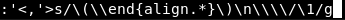Of course, I'm not smart. I didn't get this right on my first try. E.g. I didn't escape the parentheses (which you need to do to make grouping work, for some reason). So, scrolling up my s&r history to edit the command, I went one step too far back, and turns out, my backup actually saved my old s&r history. Now recall: I basically hadn't used the computer for 2 months. So, I present to you, the last s&r I performed before my 2 month extended absence. The final act. The grand finale.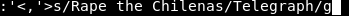Ahh, yes. Of course, of course. Took me a while to remember the context, but now I do. Good times, good times. Perhaps one day you shall see the fruits of that labor, reader. Wait, you came back?This section is titled "Part a (attempt)" because while I was writing my solution to this part, I realized it was WRONG. See below:

Looking back at 5.1, the equations of the node and cusp respectively are

 x6 + y6 - xy = 0 (Node) (1) x4 + y4 + x2 - x3 = 0(Cusp) (2)

#### Node

Let's start with the node. We can blow it up by setting

 xu = ty

where t,u are projective coordinates of P1. We can consider the open set by taking t = 1 (BTW, back in 4.10, I was wondering what about the case t = 0. I shall get back around to that later).
So we have

 y = xu

Hence, substituting into (1) turns it into

 x6 + x6u6 - x2u = 0⇔x2(x4 + x4u6 - u) = 0

x2 = 0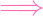x = 0 yields y = 0 and u free, which is the exceptional curve. So we instead look at

 x4 + x4u6 - u = 0 (3) y - xu = 0 (4)

which is A3
To find singularities, we look at the Jacobian, which is (ordering the coordinates as x,y,u for convenience)

 J =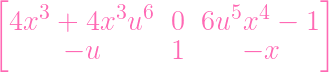(5)

Okay, first thing to note here is that if x = 0, the matrix turns into

 J =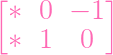(6)

which is clearly nonsingular. So let's go back to (5) and assume x0. Dividing the first row by 4x3 (remember x0), we get

 J =(7)

Now I'd like 1 + u6 to be nonzero, so I can divide by it. For the sake of contradiction, let's assume

 1 + u6 = 0 (8)

Let's see if this is possible with regard to (3):

 x4 + x4u6 - u = 0x4(1 + u6) - u = 0- x40 - u = 0 (8)- u = 0

Oh, actually, NOT a contradiction–in a good way. If we plug in u = 0 into (9), we actually get a nonsingular matrix:

 J =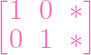So we've handled the 1 + u6 = 0 case. Now let's try 1 + u60 on (9). Now we can divide the first row and obtain

 J =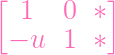(9)

Then multiplying by u on the first row and adding to the second row yields a nonsingular matrix. DONE.

....Or not. We're only done with the affine piece t0.

Dear reader, I know it has been quite a while, but do you recall how in 4.10, I expressed some confusion about whether or not I should consider the other open affine, u0?

Well, this time I looked at the solution, and it turns out I was correct to be confused. YOU NEED TO CONSIDER BOTH OPEN SETS TO COVER THE WHOLE BLOWUP (duh). But Hartshorne was abusing language and using "blowup" to denote the part of the blowup that was in t0. What a fucking confusing mess. Well, now I know.

FORTUNATELY, the node equation is symmetric about y = x:

 x6 + y6 - xy = 0

so the u0 case is essentially identical to the t0 case. Done.

#### Cusp

Again, the cusp equation is

 x4 + y4 + x2 - x3 = 0 (10)

We blow up again:

 xu = ty

Unlike the node, (10) isn't symmetric on y = x. Hence we actually have to handle the t0 and u0 cases separately.

##### u≠0

i.e. u = 1, so we get

 x = ty

Plugging this into the cusp equation, (10), we get

 y2 + t4y4 + y4 - t3y3 = 0 (11) ⇔y2(1 + t4y2 + y2 - t3y) = 0 (12) (13)

y2 = 0y = 0 yields the exceptional curve, so our blow up (in the open set u0) is

 1 + t4y2 + y2 - t3y = 0 (14) x = ty (15)

This gives the Jacobian

 J =(16)

My notes tell me that I can assume t0, so let's go ahead and verify that. If I plug in t = 0 into (18), then I get

 J =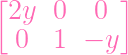(17)

This is nonsingular unless y is also 0. But if we had both t = 0 and y = 0, we'd get 1 = 0 in the cusp equation (14). Hence t = 0 is always nonsingular.
Now we can assume that t0. Looking ahead, I'd like to also assume y0. So let's handle that too (UGH).
If y = 0 (and t0), (18) turns into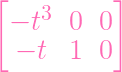And with t0, it's pretty clear that the above is nonsingular. HENCE, we can assume that both t0 and y0. Going back to (18), I can divide by t2y on the first row

 J2 =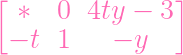(18)

(My notes also tell me that I'm ignoring that top left component)

Now as long as I show that the top right component 4t2y2 - 3ty is nonzero, I'm done. So let's suppose

 4ty - 3 = 0 ⇔ty = 3∕4

Now let's go ahead and try this on (14):

 1 + t4y2 + y2 - t3y = 0 ⇔1 + (ty)2t2 + y2 - (ty)t2 = 0 ⇔1 + (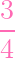)2t2 + y2 -t2 = 0

FFFFFFFFFFFFUUUUUUUUUUUUUUUUUUUUUUUUUUUUUUUUUUCCCCCCCCCCCCCCCCCCCCCCCKKKKKKKKKKKKKKKKKKK
This is where my notes stopped. My notes stopped here because in my head I didn't consider the square on the first (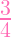), and I therefore thought the second and fourth terms cancel. THEY DON'T THOUGH. (hi /qa/). Now what? I DON'T FUCKING KNOW. FUCK PART A.

### Part b

HADTA LOOK AT THE SOLUTION FOR THIS ONE.
Assume P = (0, 0) without loss of generality (see 5.3 for the definition of multiplicity).
Here's the main trick: Letting Y = Z(f), we can assume without loss of generality that

 f(x,y) = xy + g(x,y) (19)

(where the terms of g are higher degree)
I guess this would be the canonical example of "multiplicity 2 curve with distinct tangent directions (x and y)". I suppose any homogenous quadratic with "distinct tangent directions" at the origin can be linearly transformed into xy, eh? Shall I prove it? NAY. Not today. Let's take this assumption for granted and roll with it.
Alright, now we get to blow this baby up:

 xu = ty

Let's check the open set t0 first (t = 1), so we get xu = y, and plugging this into (19) yields

 x2u + g(x,xu) = 0

Since each term in g is degree 3 or higher (in x, not just x and y, since we plugged in y = xu), we can pull out an x2 from all of f:

 x2(u + h(x,u)) = 0

Where h(x,u) is just some element in k[x,u] where every term has a factor of x (since it was degree 3 in x and we only pulled out x2. So note that h(0,u) = 0). The exceptional curve is

 x = 0 y = 0 u free

And our blowup is

 u + h(x,u) = 0 (20) y = xu (21)

Now we care about where the exceptional curve meets the blowup. So let's plug in x = 0 into (20), which gives

 u + h(0,u) = 0 ⇔u + 0 = 0 ⇔u = 0

Hence, the blowup meets the exceptional curve at one point: (0, 0, 1, 0).
Waiiiiiiiiit. One point? Just one point? The exercise says there are two. WELL, I'm "abusing language", JUST LIKE MOTHERFUCKING HARTSHRONE HIMSELF.. It's the part of the blowup in the open set t0 that meets ϕ-1(P) at that point. We still have to consider u0. BUT FIRST: Let's check that this point, (0, 0, 1, 0), is nonsingular.
Take the Jacobian of the blowup

 J =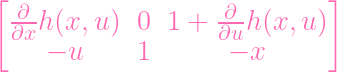We care about (0, 0, 0, 1), so x,u = 0. Actually, we only need to plug in x = 0.
Note that when taking the partial with respect to u, x is a constant. So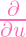h(x,u)|x=0 =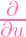h(0,u) =0 = 0. Hence, plugging in x = 0 into the Jacobian yields

 J =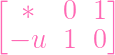which is clearly nonsingular regardless of u (in particular, for u = 0). Hence (0, 0, 1, 0) is nonsingular.
If we check the other open set, u0, we get an analogous argument (since the quadratic term xy is symmetric), showing that the other point of intersection is (0, 0, 0, 1), and it is nonsingular.
PART B DONE

### Part c

And now, the tacnode

 x2 = x4 + y4 (22)

OKAY: Here's one weird thing. The definition of ϕ actually just changed. Recall the definition of blowup: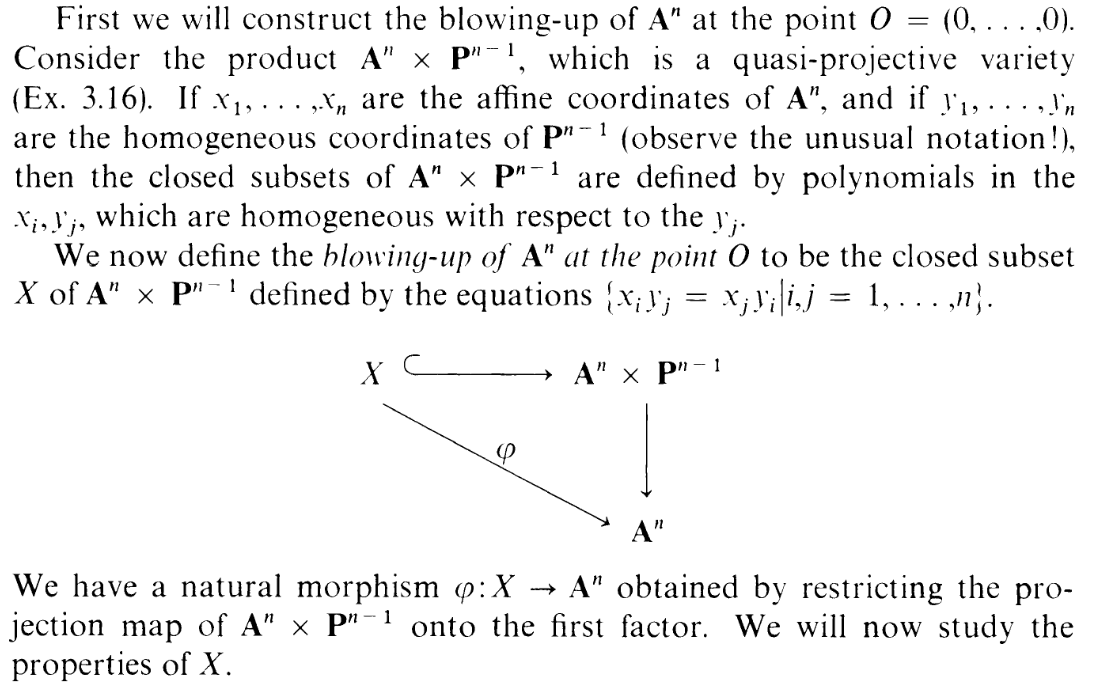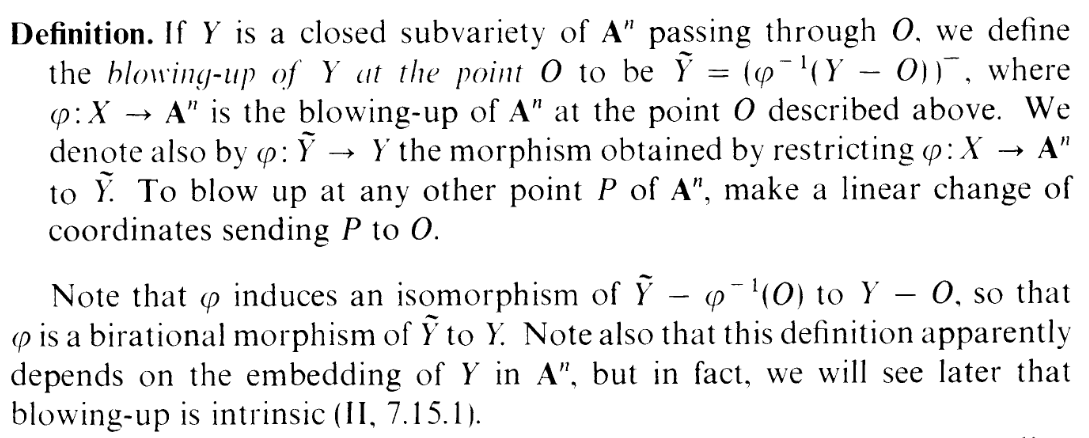In part (b), we were using the first notion of blowup: ϕ : X An, and we were checking where the exceptional curve, ϕ-1(P) intersected . For part (c), we're instead considering the restriction ϕ : Y . So this time ϕ-1(P) is the part of the exceptional curve that is inside . In other words... we're looking at the same thing again (where the blowup intersects the exceptional curve). But the phrasing is confusing. Basically, ϕ can mean different things, depending on the context.
There's another weird thing, but we'll get to that soon enough. Let's blow up:

 xu = ty

And let's look at the t0 side first, so we get xu = y, and

 x2 = x4 + x4u4 ⇔0 = x4 + x4u4 - x2 ⇔0 = x2(x2 + x2u4 - 1)

The exceptional curve is

 x = 0 y = 0 u free

And the blowup is

 x2 + x2u4 - 1 = 0y = xu

Where does (this part of) the blowup meet the exceptional curve? Well, if we plug in x = 0, we get -1 = 0. So the blowup doesn't meet ϕ-1(P) on t0 (*EDIT: ACTUALLY, FOR PART D WE DON'T CARE IF IT MEETS THE EXCEPTIONAL CURVE OR NOT. WE CARE ABOUT THE ORIGIN O IN THE NEW CURVE. WELL, x,u = 0 YIELDS -1 = 0 ANYWAY). So let's check the other open set, u0, which allows the substitution x = ty. Then our curve equation (22) turns into

 t2y2 = t4y4 + y4 ⇔0 = t4y4 + y4 - t2y2 = y2(t4y2 + y2 - t2)

Our exceptional curve is

 x = 0 y = 0 t free

And the blowup is

 t4y2 + y2 - t2 = 0 (23) x = ty (24)

in A3. Where does this meet the exceptional curve? If we plug in y = 0 into the above, we get

 t402 + 02 - t2 = 0 ⇔t2 = 0 ⇔t = 0

So this is the point (0, 0, 0, 1). In the context of this open set, u0, this is the origin (0, 0, 0) in A3.
...Wait... A3? We defined multiplicity on curves determined by single polynomials in A2. So what the fuck is a "node" in a variety determined by two equations in A3, when we didn't even define multiplicity for this situation.
Well, what does the solution say? It just ignores the x lmfao. What is the justification for this? I have no fucking clue lmfao. I mean, I was tempted to do this myself, actually. If we look back at the equations that determine (this side of) the blowup,

 t4y2 + y2 - t2 = 0 x = ty

You'll note the x part of the curve is dependent on the other two variables. So it's... not important in a sense? If we just ignore the x and just look at the "interesting part",

 t4y2 + y2 - t2 = 0

it's an A2 curve that fits the context of 5.3. And we can now clearly see it has a multiplicity of 2. And if we factor the degree 2 terms, we can write

 t4y2 + (y + t)(y - t) = 0

so the tangent directions are distinct. Hence, it's a node.

### Part d

Okay, same idea, right?

 y3 - x5 = 0

Blow up:

 xu = ty

 xu = y

So

 x3u3 - x5 = 0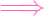x3(u3 - x2) = 0

x3 = 0 is the exceptional curve, and the blowup is

 xu = y u3 - x2 = 0

Of course, from part (b), we know that the part we care about is the second curve, and that is clearly a double point (but the tangent directions aren't different, so I guess it's a "cusp" rather than a node? Yeah, the graph says so)
Yaaaaaaaaaaaaaaay, we're done. Except... what happens in the other open set? I picked t0 first randomly. Ermm... so, let's try u0 and see what happens

 x = ty

So

 y3 - t5y3 = 0y3(1 - t5) = 0

So our blowup is

 x = ty1 - t5 = 0

Of course, we only consider the second equation, which is a set in A2 with coordinates x,t.
Okay, here's some fun confusion. Where does this curve meet the exceptional curve? Everywhere... right? Setting x,y = 0 doesn't restrict the solution set at all. But 1 - t5 doesn't have a multiplicity of 2. It has a multiplicity of 0. Ah

Note that P Y μP(Y ) > 0

The origin, P = O isn't on this curve, and for this exercise, we care only about the new origin(s).